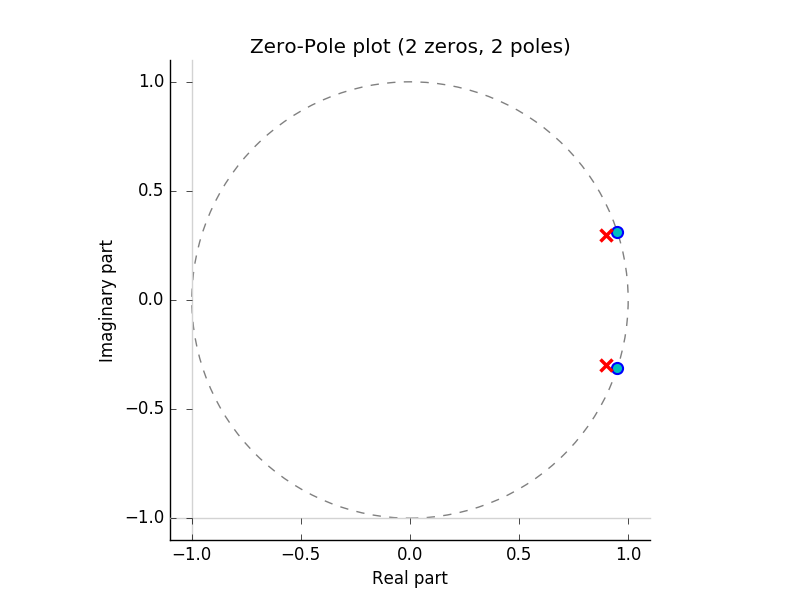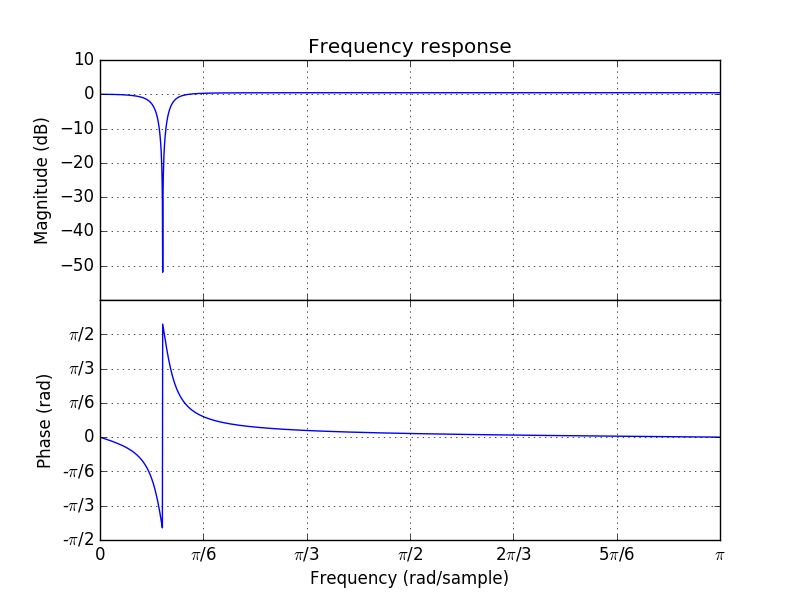# 4.2.9. The Z-Operator¶

$$\newcommand{\op}{\mathsf #1}$$ $$\newcommand{\ztarrow}{\stackrel{\op Z}{\longrightarrow}}$$ $$\newcommand{\z}{\op z}$$

The Z-transform takes a sequence $$x[n]$$ and returns a function $$X(z)$$. In the z-domain we have seen that shifting a signal $$x[n]$$ over $$n_0$$, resulting in $$x[n-n_0]$$, leads to the Z-transform $$z^{-n_0}X(z)$$ where $$X(z)$$ is the transform of the original signal.

This observation has lead to the introduction of the $$\z$$-operator that takes a discrete signal $$x$$ and shifts it:

$(\z x)[n] = x[n-1]$

With this algebraic notation of a shift, together with multiplications and summations this is all we need to characterize /all/ linear translation invariant filters.

Note that shifting over 3 samples corresponds with the operator $$\z \z \z = \z^3$$ or in general:

$(\z^{m} x)[n] = x[n-m]$

Evidently the operator $$\z^{-1}$$ corresponds with a shift in the opposite direction and thus the above equation may be interpreted for both positive and negative (integer) values of $$m$$.

A simple non-causal running average filter over 5 samples can be defined as the operator:

$\op f = \tfrac{1}{5}\left( \z^2 + \z^1 + 1 + \z^{-1} + \z^{-2}\right)$

Using this filter to process a signal $$x$$ is denoted as $$\op f x$$ or $$\op f(x)$$ where we assume $$x$$ is a discrete signal.

A recursive band stop filter is defined with:

$y[n] = 1.8 y[n-1] - 0.9 y[n-2] + x[n] - 1.9 x[n-1] + x[n-2]$

Using the $$\z$$ operator we may write:

$y[n] = 1.8 (\z^{-1} y)[n] - 0.9 (\z^{-2}y)[n] + x[n] - 1.9 (\z^{-1}x)[n] + (\z^{-2}x)[n]$

because now all signals are indexed at $$n$$ we may leave the index out to obtain the expression that is valid for all $$n$$:

$y = 1.8 \z^{-1} y - 0.9 \z^{-2}y + x - 1.9 \z^{-1}x + \z^{-2}x$

or equivalently:

$y = \frac{1 - 1.9 \z^{-1} + \z^{-2}}{1 - 1.8 \z^{-1} + 0.9 z^{-2}} x = \frac{\z^2-1.9\z+1}{\z^2-1.8\z+0.9} x$

This band stop filter thus can be formally defined as:

$\op f = \frac{\z^2-1.9\z+1}{\z^2-1.8\z+0.9}$

and of course in the definition of the filter $$\op f$$ you recognize the Z-transform of the impulse response of the filter characterized with the Z-transform:

$H(z) = \frac{z^2-1.9z+1}{z^2-1.8z+0.9}$

Please note however that $$H(z)$$ is not the filter $$\op f$$. In the expression for $$H(z)$$, $$z$$ is a complex number whereas in the expression for $$\op f$$, $$\z$$ is an operator. The operator notation for $$\op f$$ is a symbolic notation. It can be implemented in practice using the difference equation we started with.

Using the $$\z$$ operator to define discrete LTI filters is nowadays often used. In fact even software is written in which the $$\z$$ operator notation plays a central role. Below we give some examples using the ‘audiolazy’ Python package.

In : from audiolazy import z;

In : H = (z**2-1.9*z+1)/(z**2-1.8*z+0.9);

In : H.zplot().savefig('figures/hzplot.pdf');

In : H.plot().savefig('figures/hfplot.pdf');Note that in the code given here the function savefig() is used to save the figure in a file. In an interactive session you can use show() instead.

Although conceptually nice, audiolazy still lacks soms of the standard filters. A lot of these standard filters are available in scipy.signal. In the lecture notes on filter design some examples of the use of scipy will be given.